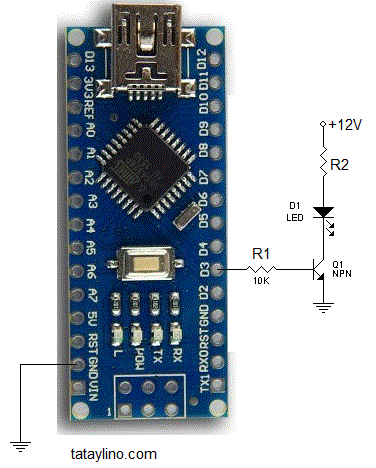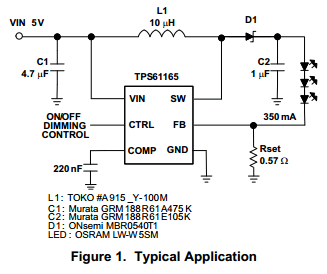# Connecting High Power LED to ArduinoArduino digital can only drive a few milliamps and up to 5V only. You can’t use it to drive a high power LED directly. If you want to drive a high power LED using Arduino, you need to use a driver.   There are 2 options, 1 is to use a transistor driver or use an IC driver. A transistor driver is the easiest option, it will only require 3 components(2 resistors and 1 transistor). The transistor is either a MOSFET or a BJT.Figure 1 – Driving LED using BJT

In the example above, we are using a NPN transistor for driving a LED. R1 is used to limit the current coming out of the Arduino. Without R1, Arduino digital pin might got damaged due to high current sinking from the output pin. The output voltage of the Arduino will be limited to the Vbe(base-emitter voltage) of Q1 without R1. While R2 limits the current for the LED.

R2 = (VDD – VLED – VCEsat)/ILED
where:
VLED – voltage of the LED
VCEsat – saturation voltage of the Q1 at ILED current
ILED – current of the LED at VLED
VDD – supply voltage(in this example: 5V)

• Note: You do not need R2 if VLED is equal to VCC. For example if voltage of the LED is 12V and your supply is also 12V, R2 will be useless.

Minimum value of R1:

R1 = (Vout – Vbe)/Iin
where:
Vin – output voltage of Arduino (usually 5V or 3.3V)
Vbe – base-emitter voltage of Q1(usually around 0.5 – 0.7V)
Iin – Max output current of Arduino ( ~10-20mA)

Max value of R1:
this is a little tricky so let’s derive the equation, shall we?

IC = Ib * β
replace Ib with equation from the circuit:
IC = ((Vin – Vbe)/R1) * β
IC = ILED
ILED = ((Vin – Vbe)/R1) * β
ILED/β = (Vin – Vbe)/R1
R1/β = (Vin – Vbe)/ILED

R1 = ((Vin – Vbe) * β)/ILED

where:
β – transister beta or HFE
ILED – LED current
Vbe – base-emitter voltage of the transistor
Vin – output voltage of arduino
IC – Collector current of the transistor

If you have computed lower maximum resistance than the minimum resistance then you should consider changing your transistor with higher HFE. You may consider using  a darlington transistor or a MOSFET.

## LED Driver IC

LED driver IC is a bit expensive but it has some advantage. It can supply a constant current which leads to more constant brightness especially if you are using multiple LED’s at a time. It also has some sort of overload protection. It is also more efficient and very ideal for battery powered applications which will have longer battery life. It is not usually simple because it is usually using switching power supply topology.Figure 2 – LED driver IC

## Dimming LED with Arduino

Dimming is done by using PWM or Pulse Width Modulation. PWM works by rapid turning on and off of the output. The brightness of the LED is controlled by controlling the ratio of the on versus the off state. PWM is easily done with Arduino, thanks to its very simple library.

Sample code:

int ledPin = 9; // LED connected to digital pin 9
int analogPin = 3; // potentiometer connected to analog pin 3
int val = 0; // variable to store the read value

void setup() {
pinMode(ledPin, OUTPUT); // sets the pin as output
}

void loop() {